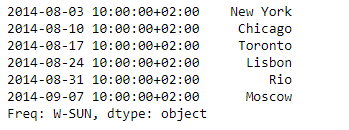Python | Pandas Series.transpose()

• Last Updated : 05 Feb, 2019

Pandas series is a One-dimensional ndarray with axis labels. The labels need not be unique but must be a hashable type. The object supports both integer- and label-based indexing and provides a host of methods for performing operations involving the index.

Pandas Series.transpose() function return the transpose, which is by definition self.

Syntax: Series.transpose(*args, **kwargs)

Parameter : None

Returns : self

Example #1: Use Series.transpose() function to find the transpose of the given Series object.

 # importing pandas as pdimport pandas as pd  # Creating the Seriessr = pd.Series(['New York', 'Chicago', 'Toronto', 'Lisbon', 'Rio', 'Moscow'])  # Create the Datetime Indexdidx = pd.DatetimeIndex(start ='2014-08-01 10:00', freq ='W',                      periods = 6, tz = 'Europe/Berlin')   # set the indexsr.index = didx  # Print the seriesprint(sr)

Output :Now we will use Series.transpose() function to find the transpose of the given series object.

 # find the transposesr.transpose()

Output :As we can see in the output, the Series.transpose() function has returned the same object as the transpose of the given series object, which is by definition self.

Example #2: Use Dataframe.transpose() function to find the transpose of the given Dataframe.

 # importing pandas as pdimport pandas as pd  # Creating the Dataframedf = pd.DataFrame({'Date':['10/2/2011', '11/2/2011', '12/2/2011', '13/2/2011'],                    'Event':['Music', 'Poetry', 'Theatre', 'Comedy'],                    'Cost':[10000, 5000, 15000, 2000]})  # Print the dataframeprint(df)

Output :Now we will use Dataframe.transpose() function to find the transpose of the given Dataframe.

 # find the transposedf.transpose()

Output :As we can see in the output, the Dataframe.transpose() function has successfully returned the transpose of the given Dataframe object.

My Personal Notes arrow_drop_up